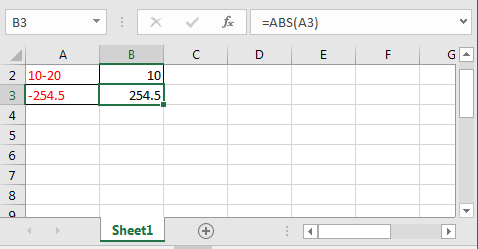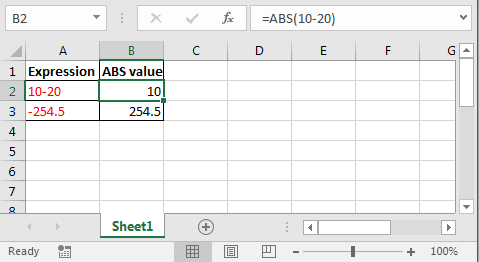# How to use the ABS function in Excel - The Absolute Value Function

The ABS function in Excel always returns positive value of an expression.Syntax of ABS formula

=ABS(number)

When and how to use ABS function?

You can use this function where values are always positive. Like distance.

Supply any expression to ABS function, which will result in number. ABS function will return distance of that number from 0 on number line. And this what absolute value means.
Example.

In Cell A2 I am subtracting 20 from 10. When i encapsulate it with ABS formula, see what we get.

=ABS(10-20)We get 10 instead of -10.
That’s it. This is how you get absolute value in Excel.

Related Articles:

Excel Convert negative number to positive number

Popular Articles:

50 Excel Shortcuts to Increase Your Productivity

How to use the VLOOKUP Function in Excel

How to use the COUNTIF in Excel 2016

How to Use SUMIF Function in Excel

Terms and Conditions of use

The applications/code on this site are distributed as is and without warranties or liability. In no event shall the owner of the copyrights, or the authors of the applications/code be liable for any loss of profit, any problems or any damage resulting from the use or evaluation of the applications/code.